The QLIM Procedure

HETERO Statement

• HETERO dependent variables ~ exogenous variables </ options > ;

The HETERO statement specifies variables that are related to the heteroscedasticity of the residuals and the way these variables are used to model the error variance. The heteroscedastic regression model supported by PROC QLIM is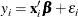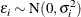See the section Heteroscedasticity for more details on the specification of functional forms.

The functional form can be specified using the LINK= option. The following option values are allowed:

EXP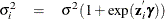LINEAR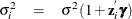When the LINK= option is not specified, the exponential link function is specified by default.

NOCONST

specifies that there be no constant in the exponential heteroscedasticity model.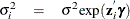SQUARE

estimates the model by using the square of linear heteroscedasticity function. For example, you can specify the following heteroscedasticity function: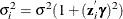model y = x1 x2 / discrete;
hetero y ~ z1 / link=linear square;


The option SQUARE does not apply to exponential heteroscedasticity function because the square of an exponential function of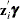is the same as the exponential of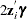. Hence the only difference is that all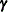estimates are divided by two.

You can use the HETERO statement within a Bayesian framework, but you should do this carefully because convergence can be slower than in the homoscedastic case. For more information see Priors for Heteroscedastic Models.